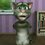# Subbu's square formula

I am here to talk about square of consecutive natural numbers . $formula=>(n+1)^2-n^2=2n+1$ suppose when we consider $8^2and9^2$ then the difference between them is 2(8)+1=16+1=17 let us check this by normal method $8^2=64;9^2=81$ the difference between them is 81-64=17 that's it i got the answer this is simple because square numbers always differ by odd numbers so 2n+1 is the general form of odd numbers and i compared and got the simple Subbu's square formula please like my note no one is liking my problems and note please if you like it nothing happens to youNote by Sudoku Subbu
6 years, 6 months ago

This discussion board is a place to discuss our Daily Challenges and the math and science related to those challenges. Explanations are more than just a solution — they should explain the steps and thinking strategies that you used to obtain the solution. Comments should further the discussion of math and science.

When posting on Brilliant:

• Use the emojis to react to an explanation, whether you're congratulating a job well done , or just really confused .
• Ask specific questions about the challenge or the steps in somebody's explanation. Well-posed questions can add a lot to the discussion, but posting "I don't understand!" doesn't help anyone.
• Try to contribute something new to the discussion, whether it is an extension, generalization or other idea related to the challenge.

MarkdownAppears as
*italics* or _italics_ italics
**bold** or __bold__ bold
- bulleted- list
• bulleted
• list
1. numbered2. list
1. numbered
2. list
Note: you must add a full line of space before and after lists for them to show up correctly
paragraph 1paragraph 2

paragraph 1

paragraph 2

[example link](https://brilliant.org)example link
> This is a quote
This is a quote
    # I indented these lines
# 4 spaces, and now they show
# up as a code block.

print "hello world"
# I indented these lines
# 4 spaces, and now they show
# up as a code block.

print "hello world"
MathAppears as
Remember to wrap math in $$ ... $$ or $ ... $ to ensure proper formatting.
2 \times 3 $2 \times 3$
2^{34} $2^{34}$
a_{i-1} $a_{i-1}$
\frac{2}{3} $\frac{2}{3}$
\sqrt{2} $\sqrt{2}$
\sum_{i=1}^3 $\sum_{i=1}^3$
\sin \theta $\sin \theta$
\boxed{123} $\boxed{123}$

Sort by:

This is the general form of odd numbers.This was found before itself.This is not Subbu's square formula.

- 6 years, 6 months ago

liked it

- 5 years, 9 months ago

This is a good note i am inspired by you

- 6 years, 6 months ago

thank you my boy

- 6 years, 6 months ago